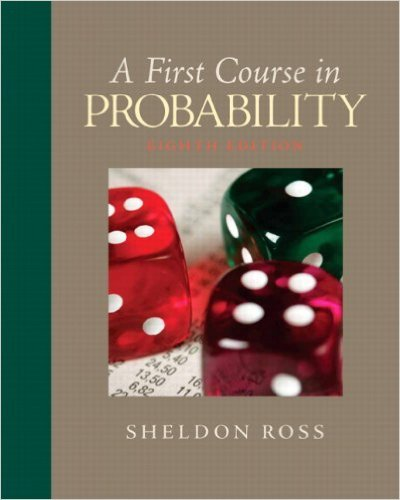×
Get Full Access to First Course In Probability - 8 Edition - Chapter 5 - Problem 5.39
Get Full Access to First Course In Probability - 8 Edition - Chapter 5 - Problem 5.39

×

# If X is an exponential random variable withparameter = 1,ISBN: 9780136033134 163

## Solution for problem 5.39 Chapter 5

First Course in Probability | 8th Edition

• Textbook Solutions
• 2901 Step-by-step solutions solved by professors and subject experts
• Get 24/7 help from StudySoup virtual teaching assistantsFirst Course in Probability | 8th Edition

4 5 1 320 Reviews
13
1
Problem 5.39

If X is an exponential random variable withparameter = 1, compute the probability densityfunction of the random variable Y defined byY = logX.

Step-by-Step Solution:
Step 1 of 3

Color Coding: Topics MINITAB Examples STT 351 Notes Normal −1 2 f x = 1 e 2 x ( ) 2Π ­inf < x < inf √ To find probabilities between two points (a,b] we have to integrate this function between a,b. Using MINITAB: 1) Calc 2) Probability distribution 3) Normal (mean = 0, Standard deviation = 1) 4) Select cumulative probability This will give the area under the curve up to the point x For any a value, the area under the curve up to the point a is = P(X ≤ a) # If we want to find the area under the curve between two points we can use the equation….. P( a < X ≤ b ) = P( X ≤ b ) – P( X ≤ a ) Using MINITAB: To find

Step 2 of 3

Step 3 of 3

##### ISBN: 9780136033134

The full step-by-step solution to problem: 5.39 from chapter: 5 was answered by , our top Statistics solution expert on 11/23/17, 05:06AM. This full solution covers the following key subjects: variable, random, logx, Exponential, function. This expansive textbook survival guide covers 10 chapters, and 495 solutions. This textbook survival guide was created for the textbook: First Course in Probability, edition: 8. First Course in Probability was written by and is associated to the ISBN: 9780136033134. Since the solution to 5.39 from 5 chapter was answered, more than 232 students have viewed the full step-by-step answer. The answer to “If X is an exponential random variable withparameter = 1, compute the probability densityfunction of the random variable Y defined byY = logX.” is broken down into a number of easy to follow steps, and 23 words.

Unlock Textbook Solution